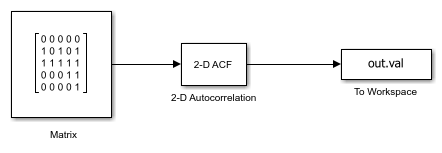# Compute Autocorrelation of Input Matrix

This example shows how to compute autocorrelation of a 5-by-5 input matrix using the `2-D` `Autocorrelation` block.

The output of the model is a 9-by-9 matrix consisting of autocorrelation coefficients.

The coefficient values shows the similarity between the input matrix and its shifted form. The value of the autocorrelation coefficient at a point (`i`, `j`) is high, if the values in the original matrix and the shifted matrix are similar.

```model = 'ex_blkautocorrelation.slx'; open_system(model) ```Run the model and export the computed autocorrelation coefficients to MATLAB workspace. Display the coefficients using `disp` function.

```out = sim(model); disp(out.val) ```
``` 0 0 0 0 0 0 0 0 0 1 0 1 0 1 0 0 0 0 2 2 2 2 2 1 0 0 0 2 3 4 5 6 3 2 1 1 2 2 5 5 11 5 5 2 2 1 1 2 3 6 5 4 3 2 0 0 0 1 2 2 2 2 2 0 0 0 0 1 0 1 0 1 0 0 0 0 0 0 0 0 0 ```